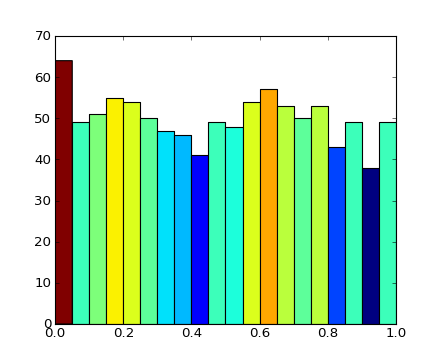# pylab_examples example code: hist_colormapped.py¶```import numpy as n
from pylab import figure, show
import matplotlib.cm as cm
import matplotlib.colors as colors

fig = figure()
Ntotal = 1000
N, bins, patches = ax.hist(n.random.rand(Ntotal), 20)

#I'll color code by height, but you could use any scalar

# we need to normalize the data to 0..1 for the full
# range of the colormap
fracs = N.astype(float)/N.max()
norm = colors.normalize(fracs.min(), fracs.max())

for thisfrac, thispatch in zip(fracs, patches):
color = cm.jet(norm(thisfrac))
thispatch.set_facecolor(color)

show()
```

Keywords: python, matplotlib, pylab, example, codex (see Search examples)